Search by Topic

Resources tagged with Interactivities similar to The Lily Pond:

Filter by: Content type:
Age range:
Challenge level:

There are 154 results

Broad Topics > Information and Communications Technology > InteractivitiesCode Breaker

Age 7 to 11 Challenge Level:

This problem is based on a code using two different prime numbers less than 10. You'll need to multiply them together and shift the alphabet forwards by the result. Can you decipher the code?Fractions and Percentages Card Game

Age 11 to 16 Challenge Level:

Match the cards of the same value.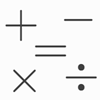Which Symbol?

Age 7 to 11 Challenge Level:

Choose a symbol to put into the number sentence.Coded Hundred Square

Age 7 to 11 Challenge Level:

This 100 square jigsaw is written in code. It starts with 1 and ends with 100. Can you build it up?Junior Frogs

Age 5 to 11 Challenge Level:

Have a go at this well-known challenge. Can you swap the frogs and toads in as few slides and jumps as possible?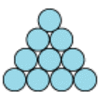Difference

Age 7 to 11 Challenge Level:

Place the numbers 1 to 10 in the circles so that each number is the difference between the two numbers just below it.A Square of Numbers

Age 7 to 11 Challenge Level:

Can you put the numbers 1 to 8 into the circles so that the four calculations are correct?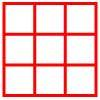Age 5 to 11 Challenge Level:

Place six toy ladybirds into the box so that there are two ladybirds in every column and every row.Domino Numbers

Age 7 to 11 Challenge Level:

Can you see why 2 by 2 could be 5? Can you predict what 2 by 10 will be?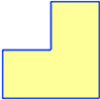Twice as Big?

Age 7 to 11 Challenge Level:

Investigate how the four L-shapes fit together to make an enlarged L-shape. You could explore this idea with other shapes too.One Million to Seven

Age 7 to 11 Challenge Level:

Start by putting one million (1 000 000) into the display of your calculator. Can you reduce this to 7 using just the 7 key and add, subtract, multiply, divide and equals as many times as you like?More Transformations on a Pegboard

Age 7 to 11 Challenge Level:

Use the interactivity to find all the different right-angled triangles you can make by just moving one corner of the starting triangle.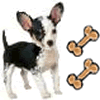Ratio Pairs 3

Age 11 to 16 Challenge Level:

Match pairs of cards so that they have equivalent ratios.Treasure Hunt

Age 7 to 14 Challenge Level:

Can you find a reliable strategy for choosing coordinates that will locate the treasure in the minimum number of guesses?Arrangements

Age 7 to 11 Challenge Level:

Is it possible to place 2 counters on the 3 by 3 grid so that there is an even number of counters in every row and every column? How about if you have 3 counters or 4 counters or....?Square Coordinates

Age 11 to 14 Challenge Level:

A tilted square is a square with no horizontal sides. Can you devise a general instruction for the construction of a square when you are given just one of its sides?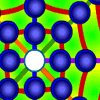Counter Roundup

Age 7 to 11 Challenge Level:

A game for 1 or 2 people. Use the interactive version, or play with friends. Try to round up as many counters as possible.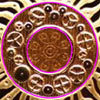Coin Cogs

Age 7 to 11 Challenge Level:

Can you work out what is wrong with the cogs on a UK 2 pound coin?Isosceles Triangles

Age 11 to 14 Challenge Level:

Draw some isosceles triangles with an area of $9$cm$^2$ and a vertex at (20,20). If all the vertices must have whole number coordinates, how many is it possible to draw?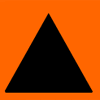Sorting Symmetries

Age 7 to 11 Challenge Level:

Find out how we can describe the "symmetries" of this triangle and investigate some combinations of rotating and flipping it.Square It

Age 11 to 16 Challenge Level:

Players take it in turns to choose a dot on the grid. The winner is the first to have four dots that can be joined to form a square.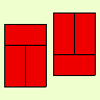Fault-free Rectangles

Age 7 to 11 Challenge Level:

Find out what a "fault-free" rectangle is and try to make some of your own.Nine-pin Triangles

Age 7 to 11 Challenge Level:

How many different triangles can you make on a circular pegboard that has nine pegs?Triangles All Around

Age 7 to 11 Challenge Level:

Can you find all the different triangles on these peg boards, and find their angles?Teddy Town

Age 5 to 14 Challenge Level:

There are nine teddies in Teddy Town - three red, three blue and three yellow. There are also nine houses, three of each colour. Can you put them on the map of Teddy Town according to the rules?Instant Insanity

Age 11 to 18 Challenge Level:

Given the nets of 4 cubes with the faces coloured in 4 colours, build a tower so that on each vertical wall no colour is repeated, that is all 4 colours appear.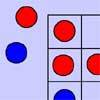Red Even

Age 7 to 11 Challenge Level:

You have 4 red and 5 blue counters. How many ways can they be placed on a 3 by 3 grid so that all the rows columns and diagonals have an even number of red counters?Konigsberg Plus

Age 11 to 14 Challenge Level:

Euler discussed whether or not it was possible to stroll around Koenigsberg crossing each of its seven bridges exactly once. Experiment with different numbers of islands and bridges.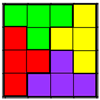Tetrafit

Age 7 to 11 Challenge Level:

A tetromino is made up of four squares joined edge to edge. Can this tetromino, together with 15 copies of itself, be used to cover an eight by eight chessboard?Colour in the Square

Age 7 to 16 Challenge Level:

Can you put the 25 coloured tiles into the 5 x 5 square so that no column, no row and no diagonal line have tiles of the same colour in them?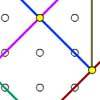Coordinate Tan

Age 7 to 11 Challenge Level:

What are the coordinates of the coloured dots that mark out the tangram? Try changing the position of the origin. What happens to the coordinates now?Age 7 to 11 Challenge Level:

Three beads are threaded on a circular wire and are coloured either red or blue. Can you find all four different combinations?Take the Right Angle

Age 7 to 11 Challenge Level:

How many times in twelve hours do the hands of a clock form a right angle? Use the interactivity to check your answers.Counters

Age 7 to 11 Challenge Level:

Hover your mouse over the counters to see which ones will be removed. Click to remove them. The winner is the last one to remove a counter. How you can make sure you win?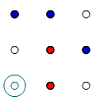Coordinate Cunning

Age 7 to 11 Challenge Level:

A game for two people that can be played with pencils and paper. Combine your knowledge of coordinates with some strategic thinking.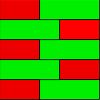Combining Cuisenaire

Age 7 to 11 Challenge Level:

Can you find all the different ways of lining up these Cuisenaire rods?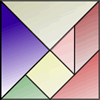Age 7 to 11 Challenge Level:

How can the same pieces of the tangram make this bowl before and after it was chipped? Use the interactivity to try and work out what is going on!Seven Flipped

Age 7 to 11 Challenge Level:

Investigate the smallest number of moves it takes to turn these mats upside-down if you can only turn exactly three at a time.Magic Potting Sheds

Age 11 to 14 Challenge Level:

Mr McGregor has a magic potting shed. Overnight, the number of plants in it doubles. He'd like to put the same number of plants in each of three gardens, planting one garden each day. Can he do it?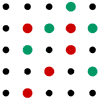Seeing Squares for Two

Age 5 to 11 Challenge Level:

Seeing Squares game for an adult and child. Can you come up with a way of always winning this game?Four Triangles Puzzle

Age 5 to 11 Challenge Level:

Cut four triangles from a square as shown in the picture. How many different shapes can you make by fitting the four triangles back together?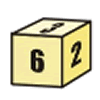First Connect Three for Two

Age 7 to 11 Challenge Level:

First Connect Three game for an adult and child. Use the dice numbers and either addition or subtraction to get three numbers in a straight line.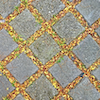Seeing Squares

Age 5 to 11 Challenge Level:

Players take it in turns to choose a dot on the grid. The winner is the first to have four dots that can be joined to form a square.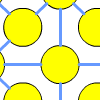One to Fifteen

Age 7 to 11 Challenge Level:

Can you put the numbers from 1 to 15 on the circles so that no consecutive numbers lie anywhere along a continuous straight line?Estimating Angles

Age 7 to 14 Challenge Level:

How good are you at estimating angles?Multiples Grid

Age 7 to 11 Challenge Level:

What do the numbers shaded in blue on this hundred square have in common? What do you notice about the pink numbers? How about the shaded numbers in the other squares?Dotty Circle

Age 7 to 11 Challenge Level:

Watch this film carefully. Can you find a general rule for explaining when the dot will be this same distance from the horizontal axis?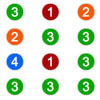Winning the Lottery

Age 7 to 11 Challenge Level:

Try out the lottery that is played in a far-away land. What is the chance of winning?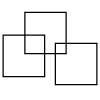Three Squares

Age 5 to 11 Challenge Level:

What is the greatest number of squares you can make by overlapping three squares?